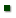Skip to main content
ARS Home » Research » Publications at this Location » Publication #166433

Title: METABOLIZABLE PROTEIN REQUIREMENTS FOR MAINTENANCE AND GAIN OF GROWING GOATS

AuthorLUO, JGOETSCH, ANSAHLAI, ISAHLU, TFerrell, CalvinOWENS, FGALYEAN, MMOORE, JJOHNSON, Z

 Submitted to: Small Ruminant Research Publication Type: Peer Reviewed Journal Publication Acceptance Date: 4/5/2004 Publication Date: 7/1/2004 Citation: Luo, J., Goetsch, A.L., Nsahlai, I.V., Sahlu, T., Ferrell, C.L., Owens, F.N., Galyean, M.L., Moore, J.E., Johnson, Z.B. 2004. Metabolizable protein requirements for maintenance and gain of growing goats. Small Ruminant Research. 53:309-326. Interpretive Summary: Using a database of treatment mean observations from reports with growing goats, along with feedstuff crude protein degradation properties, metabolizable protein (MP) requirements were determined by regressing MP intake (MPI) against average daily gain (ADG). The MP requirement for maintenance was 3.07 g/kg BW0.75, and the MP requirement for body weight (BW) gain of meat goats was 0.404 g/g ADG and that for dairy and indigenous goats was 0.290 g/g ADG. Because of the large number of observations on which these estimates are based, they seem useful in determining diet composition for growing goats as well as predicting performance. However, because MPI may not have in all instances been more limiting to growth than metabolizable energy intake, it seems appropriate to consider the estimates as maximum requirements rather than averages and, relatedly, that supplying additional MPI as a safety factor is unwarranted. Technical Abstract: A database of 349 treatment mean observations, representing 3404 goats from 73 publications between 1973 and 2003, was used to determine metabolizable protein (MP) requirements for maintenance (MPm) and growth (MPg) of goats. Published CP degradation properties of feedstuffs and proportions of dietary ingredients were used to estimate MP intake (MPI, g/day), which was regressed against ADG, with both variables scaled by BW0.75. Goats were classified as meat (>/_50% Boer; 60 observations), dairy (selected for milk production; 129 observations) and indigenous (160 observations) biotypes. Because of differences (P < 0.01) among biotypes in slopes, separate regressions were initially performed'meat: MPI = 2.55 (S.E. = 0.360) + (0.441 (S.E. = 0.0276) x ADG) (n = 58; R2 = 0.82); dairy: MPI = 2.83 (S.E. = 0.344) + (0.299 (S.E. = 0.0238) x ADG) (n = 123; R2 = 0.57); and indigenous: MPI = 3.23 (S.E. = 0.212) + (0.281 (S.E. = 0.0304) x ADG) (n = 152; R2 = 0.36). Intercepts did not differ among biotypes (P = 0.37), but the slope for meat goats differed (P < 0.01) from those for dairy and indigenous goats; therefore, data sets for dairy and indigenous goats were pooled and split into development (n = 150) and evaluation (n = 125) subsets. Using the equation derived from the development data subset for dairy and indigenous goats (i.e., MPI = 3.14 (S.E. = 0.189) + (0.285 (S.E. = 0.0168) x ADG) (n = 144; R2 = 0.67)), MPI for the evaluation subset was predicted; regressing observed against predicted MPI of the evaluation data subset resulted in an intercept and slope not different from 0 and 1, respectively (P > 0.05). The equation from the development subset for dairy and indigenous goats was compared with the equation from the meat goat data set; there was a difference (P < 0.01) in slopes but not in intercepts (P = 0.25). Therefore, a dummy variable (D = 1 for meat goats and 0 otherwise) was used to develop a common intercept equation: MPI = 3.07 (S.E. = 0.165) + (0.290 (S.E. = 0.0150) x ADG) + (0.114 (S.E. = 0.0162) x D x ADG) (n = 202; R2 = 0.75). In conclusion, based on regression of MPI against ADG, MPm was 3.07 g/kg BW0.75 for all biotypes of growing goats, and MPg was 0.404 and 0.290 g/g ADG for meat and other (dairy and indigenous) goats, respectively.